The REG Procedure
 Criteria Used in Model-Selection Methods

When many significance tests are performed, each at a level of, for example, 5%, the overall probability of rejecting at least one true null hypothesis is much larger than 5%. If you want to guard against including any variables that do not contribute to the predictive power of the model in the population, you should specify a very small SLE= significance level for the FORWARD and STEPWISE methods and a very small SLS= significance level for the BACKWARD and STEPWISE methods.

In most applications, many of the variables considered have some predictive power, however small. If you want to choose the model that provides the best prediction computed using the sample estimates, you need only to guard against estimating more parameters than can be reliably estimated with the given sample size, so you should use a moderate significance level, perhaps in the range of 10% to 25%.

In addition to, the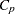statistic is displayed for each model generated in the model-selection methods. Thestatistic is proposed by Mallows (1973) as a criterion for selecting a model. It is a measure of total squared error defined as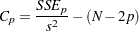where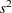is the MSE for the full model, and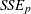is the sum-of-squares error for a model withparameters including the intercept, if any. Ifis plotted against, Mallows recommends the model wherefirst approaches. When the right model is chosen, the parameter estimates are unbiased, and this is reflected innear. For further discussion, refer to Daniel and Wood (1980).

The adjustedstatistic is an alternative tothat is adjusted for the number of parameters in the model. The adjustedstatistic is calculated as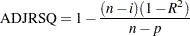whereis the number of observations used in fitting the model, andis an indicator variable that is 1 if the model includes an intercept, and 0 otherwise.Previous Page | Next Page | Top of Page